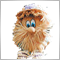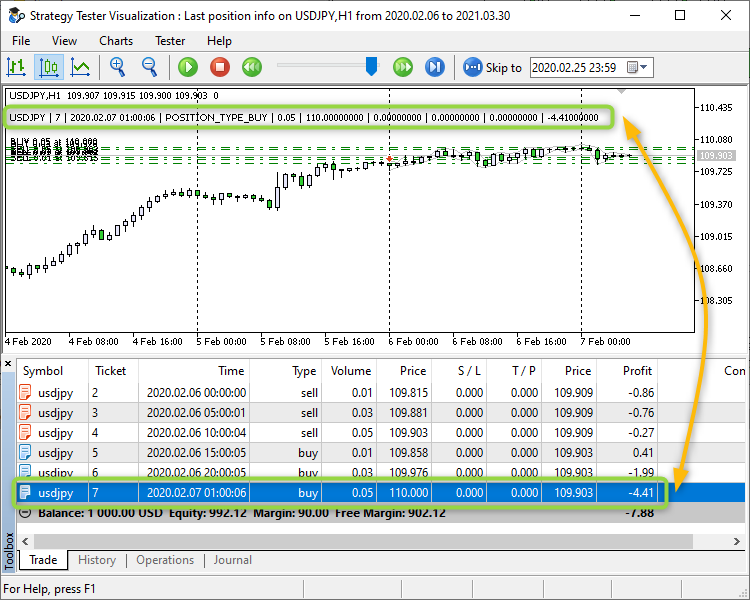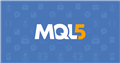Moderator
306178

RZAMK :

***

" A position cannot be reversed in the hedging system. In this case, the current position is closed and a new one with the remaining volume is opened. "

Is there any way to disable it so that I can simultaneously hold open Buy & Sell positions on a currency pair?

I think you need to ask your question on the forum - here is a thread for those who ask questions about PROGRAMMING in MQL5, but you generally have zero knowledge. You manage to misinterpret the documentation. Please do not post any more in this thread - this thread is for those who program.Moderator
306178

Example - there is a line (Horizontal line or Trend line) and you need to track when the price crosses the line.

To do this, you first need to get the price of the line - but first of all we check if there is an object with that name at all? If there is such an object, then, depending on the type of object, we get the price:

```   if(ObjectFind(ChartID(),InpObjectName)<0)
return;
double object_price=0.0;
long object_type=ObjectGetInteger(ChartID(),InpObjectName,OBJPROP_TYPE);
if(object_type==OBJ_HLINE || object_type==OBJ_TREND)
{
if(object_type==OBJ_HLINE) // OBJ_HLINE
{
object_price=ObjectGetDouble(ChartID(),InpObjectName,OBJPROP_PRICE);
}
else // OBJ_TREND
{
object_price=ObjectGetValueByTime(ChartID(),InpObjectName,TimeCurrent(),0);
}
if(object_price==0.0)
return;
MqlRates rates[];
ArraySetAsSeries(rates,true);
int start_pos=0,count=3;
if(CopyRates(Symbol(),Period(),start_pos,count,rates)!=count)
return;
if(rates.low<object_price && rates.high>object_price)
m_need_delete_all=true;
}```Moderator
306178

### Calculate positions for all symbols

Code: 'Calculate positions for all symbols.mq5

Objective: Several positions are open in the market. You need to get the number of positions (both BUY and SELL) for each symbol.

Suggested solution: the 'STRUCT_CALCULATE_POSITIONS' structure

```//+------------------------------------------------------------------+
//| Structure Calculate Positions                                    |
//+------------------------------------------------------------------+
struct STRUCT_CALCULATE_POSITIONS
{
string            symbol;                 // position symbol
int               count_sells;            // count position SELL
//--- Constructor
STRUCT_CALCULATE_POSITIONS()
{
symbol                     = "";
count_sells                = 0;
}
};```

is created and this structure is filled in the 'CalculateAllPositions'

```//+------------------------------------------------------------------+
//| Calculate all positions Buy and Sell                             |
//+------------------------------------------------------------------+
void CalculateAllPositions(STRUCT_CALCULATE_POSITIONS &SCalculatePositions[])
{
ArrayFree(SCalculatePositions);
for(int i=PositionsTotal()-1; i>=0; i--)
if(m_position.SelectByIndex(i)) // selects the position by index for further access to its properties
{
string pos_symbol=m_position.Symbol();
int size=ArraySize(SCalculatePositions);
int find=false;
for(int j=0; j<size; j++)
{
if(SCalculatePositions[j].symbol==pos_symbol)
{
if(m_position.PositionType()==POSITION_TYPE_SELL)
SCalculatePositions[j].count_sells=SCalculatePositions[j].count_sells+1;
find=true;
break;
}
}
if(!find)
{
ArrayResize(SCalculatePositions,size+1);
SCalculatePositions[size].symbol=pos_symbol;
if(m_position.PositionType()==POSITION_TYPE_SELL)
SCalculatePositions[size].count_sells=SCalculatePositions[size].count_sells+1;
}
}
//---
return;
}```

function.

For example, a test advisor has been created: first, it opens four positions and then (after calling 'CalculateAllPositions') unpacks the 'STRUCT_CALCULATE_POSITIONS' structure'

Result:

```       [symbol] [count_buys] [count_sells]
 "EURUSD"            1             1
 "EURPLN"            1             0
 "USDJPY"            1             0```
Files:Moderator
306178

### No more than N positions for each symbol Simple

Another example of how to use the 'STRUCT_CALCULATE_POSITIONS' structure. The EA opens a position on a new bar. There is no Stop Loss or Take Profit. Nobody closes open positions - this is a demonstration of how to use and how to work with the structure 'STRUCT_CALCULATE_POSITIONS'.

```//+------------------------------------------------------------------+
//| Expert tick function                                             |
//+------------------------------------------------------------------+
void OnTick()
{
STRUCT_CALCULATE_POSITIONS SCPos[];
CalculateAllPositions(SCPos);
int size=ArraySize(SCPos);
//--- Symbol 0
if(m_symbol_0)
{
datetime time_0=iTime(InpSymbol_0,Period(),0);
if(time_0!=m_prev_bars_0)
{
m_prev_bars_0=time_0;
//--- search for trading signals only at the time of the birth of new bar
for(int i=0; i<size; i++)
{
if(SCPos[i].symbol==InpSymbol_0)
{
count_sells=SCPos[i].count_sells;
break;
}
}
{
double lot=SymbolInfoDouble(InpSymbol_0,SYMBOL_VOLUME_MIN);
if(lot>0.0)
{
MqlRates rates[];
ArraySetAsSeries(rates,true);
int start_pos=0,count=3;
if(CopyRates(InpSymbol_0,Period(),start_pos,count,rates)==count)
{
if(rates.open<rates.close)
else
}
}
}
}
}
//--- Symbol 1
if(m_symbol_1)
{
datetime time_0=iTime(InpSymbol_1,Period(),0);
if(time_0!=m_prev_bars_1)
{
m_prev_bars_1=time_0;
//--- search for trading signals only at the time of the birth of new bar
for(int i=0; i<size; i++)
{
if(SCPos[i].symbol==InpSymbol_1)
{
count_sells=SCPos[i].count_sells;
break;
}
}
{
double lot=SymbolInfoDouble(InpSymbol_1,SYMBOL_VOLUME_MIN);
if(lot>0.0)
{
MqlRates rates[];
ArraySetAsSeries(rates,true);
int start_pos=0,count=3;
if(CopyRates(InpSymbol_1,Period(),start_pos,count,rates)==count)
{
if(rates.open<rates.close)
else
}
}
}
}
}
//--- Symbol 2
if(m_symbol_2)
{
datetime time_0=iTime(InpSymbol_2,Period(),0);
if(time_0!=m_prev_bars_2)
{
m_prev_bars_2=time_0;
//--- search for trading signals only at the time of the birth of new bar
for(int i=0; i<size; i++)
{
if(SCPos[i].symbol==InpSymbol_2)
{
count_sells=SCPos[i].count_sells;
break;
}
}
{
double lot=SymbolInfoDouble(InpSymbol_2,SYMBOL_VOLUME_MIN);
if(lot>0.0)
{
MqlRates rates[];
ArraySetAsSeries(rates,true);
int start_pos=0,count=3;
if(CopyRates(InpSymbol_2,Period(),start_pos,count,rates)==count)
{
if(rates.open<rates.close)
else
}
}
}
}
}
//--- Symbol 3
if(m_symbol_3)
{
datetime time_0=iTime(InpSymbol_3,Period(),0);
if(time_0!=m_prev_bars_3)
{
m_prev_bars_3=time_0;
//--- search for trading signals only at the time of the birth of new bar
for(int i=0; i<size; i++)
{
if(SCPos[i].symbol==InpSymbol_3)
{
count_sells=SCPos[i].count_sells;
break;
}
}
{
double lot=SymbolInfoDouble(InpSymbol_3,SYMBOL_VOLUME_MIN);
if(lot>0.0)
{
MqlRates rates[];
ArraySetAsSeries(rates,true);
int start_pos=0,count=3;
if(CopyRates(InpSymbol_3,Period(),start_pos,count,rates)==count)
{
if(rates.open<rates.close)
else
}
}
}
}
}
}```
Files:5

Hello
I want an indicator shows top and bottom for any time frame which write a comment beside top and bottomModerator
306178

samerfx111 :
Hello
I want an indicator shows top and bottom for any time frame which write a comment beside top and bottom

I didn't understand anything at all. What's the top? What's the bottom? Ask your question in the form of a picture.Moderator
306178

### Search for the most recent position and display information about it.

The code: 'Last position info.mq5'

```//+------------------------------------------------------------------+
//|                                           Last position info.mq5 |
//+------------------------------------------------------------------+
#property version   "1.000"
/*
*/
//---
CPositionInfo  m_position;                   // object of CPositionInfo class
//+------------------------------------------------------------------+
//| Enum Magic                                                       |
//+------------------------------------------------------------------+
enum ENUM_MAGIC
{
all_magics=0,     // All magic numbers
one_magic=1,      // One magic number
};
//+------------------------------------------------------------------+
//| Enum Symbol                                                      |
//+------------------------------------------------------------------+
enum ENUM_SYMBOL
{
all_symbols=0,    // All symbols
one_symbol=1,     // One symbol
};
//--- input parameters
input ENUM_SYMBOL          InpSymbolAllOrOne          = all_symbols; // Symbol: All OR One
input string               InpSymbolVolumeAllOrOne    = "EURUSD";    // Value for "Symbol" (only for 'Symbol')
input ENUM_MAGIC           InpMagicAllOrOne           = all_magics;  // Magic number: All OR One
input ulong                InpMagicVolumeAllOrOne     = 200;         // Value for "Magic number" (only for 'One magic numbers')
//+------------------------------------------------------------------+
//| Expert initialization function                                   |
//+------------------------------------------------------------------+
int OnInit()
{
//---
return(INIT_SUCCEEDED);
}
//+------------------------------------------------------------------+
//| Expert deinitialization function                                 |
//+------------------------------------------------------------------+
void OnDeinit(const int reason)
{
//---
Comment("");
}
//+------------------------------------------------------------------+
//| Expert tick function                                             |
//+------------------------------------------------------------------+
void OnTick()
{
//---
ENUM_POSITION_TYPE   last_pos_type  = WRONG_VALUE;
ulong                last_pos_time  = 0;            // "0" -> D'1970.01.01 00:00';
string               text           = "";
//---
for(int i=PositionsTotal()-1; i>=0; i--) // returns the number of current positions
if(m_position.SelectByIndex(i)) // selects the position by index for further access to its properties
if((InpSymbolAllOrOne==one_symbol && m_position.Symbol()==InpSymbolVolumeAllOrOne) || (!InpSymbolAllOrOne))
if((InpMagicAllOrOne==one_magic && m_position.Magic()==InpMagicVolumeAllOrOne) || (!InpMagicAllOrOne))
{
ulong pos_time=m_position.TimeMsc();
if(pos_time>last_pos_time)
{
last_pos_type=m_position.PositionType();
last_pos_time=pos_time;
//---
text="\n"+
m_position.Symbol()+
" | "+IntegerToString(m_position.Ticket())+
" | "+TimeToString(m_position.Time(),TIME_DATE|TIME_MINUTES|TIME_SECONDS)+
" | "+EnumToString(m_position.PositionType())+
" | "+DoubleToString(m_position.Volume(),2)+
" | "+DoubleToString(m_position.PriceOpen(),8)+
" | "+DoubleToString(m_position.StopLoss(),8)+
" | "+DoubleToString(m_position.TakeProfit(),8)+
" | "+DoubleToString(m_position.Swap(),8)+
" | "+DoubleToString(m_position.Profit(),8);
}
}
if(text!="")
Comment(text);
}
//+------------------------------------------------------------------+
```

Result:Files:Moderator
306178

Working with the OBJ_VLINE graphic object

Task: find the OBJ_VLINE object and get the time of the object.

There are several stages of checks and protections in the code. Stage one - searching for an object by name. Stage two - we get the type of object. The third stage is without checking, we just get the time of the object.

```//+------------------------------------------------------------------+
//|                                               OBJ_VLINE Info.mq5 |
//+------------------------------------------------------------------+
#property version   "1.000"
#property script_show_inputs
//--- input parameters
input string   InpName  = "VLine";  // VLine Name
//+------------------------------------------------------------------+
//| Script program start function                                    |
//+------------------------------------------------------------------+
void OnStart()
{
//---
long chart_id=ChartID();
if(ObjectFind(chart_id,InpName)<0)
{
}
else
{
long obj_type=ObjectGetInteger(chart_id,InpName,OBJPROP_TYPE);
if(obj_type!=OBJ_VLINE)
{
Print("ERROR: object ('",InpName,"') is not a 'OBJ_VLINE' - object is ",EnumToString((ENUM_OBJECT)obj_type));
}
else
{
datetime obj_time=(datetime)ObjectGetInteger(chart_id,InpName,OBJPROP_TIME);
Print("OBJ_VLINE '",InpName,"' time: ",TimeToString(obj_time,TIME_DATE|TIME_SECONDS));
}
}
}
//+------------------------------------------------------------------+
```Documentation on MQL5: Constants, Enumerations and Structures / Objects Constants / Object Types / OBJ_VLINE
• www.mql5.com
OBJ_VLINE - Object Types - Objects Constants - Constants, Enumerations and Structures - MQL5 Reference - Reference on algorithmic/automated trading language for MetaTrader 5
Files:168